# Fractions on the Praxis Core Math Test: Part Two

###
Announcement! As of September 2019, the content of the Praxis Core mathematics test has changed. Learn more here.
###

In the previous post, we discussed the basics of fractions as well as fraction addition and subtraction.  As promised, in this post, we will discuss fraction multiplication and division.  Have courage, friends!  With study and practice, you can become a pro with fractions!## Multiplication

The rule for multiplying fractions is very intuitive.  We just multiply across, numerator times numerator over denominator times denominator: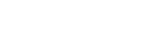The “why” here gets very interesting.  It turns out that this rule, multiplying across, is just one of the many things we are allowed to do.  A mathematician looking at (2/7) times (3/5) would see a product of four different factors: (2), (1/7), (3), and (1/5).  These four factors can be rearranged in any orders and groups, according to the Commutative and Associative laws.  Thus, in general,Yes, it is perfectly fine to multiply across, and it’s also important to recognize these many other arrangements as entirely mathematically equivalent.

## Division

Dividing fractions is also relatively easy.  Dividing by a fraction is the the same a multiplying by the reciprocal of the fraction.  The reciprocal of the fraction is the fraction “flipped over”: the old numerator becomes the denominator, and the old denominator becomes the numerator.  For example,

3/5 and 5/3 are reciprocal

2/7 and 7/2 are reciprocals

The product of any fraction times its reciprocal is 1.  The technical way to say that is that the reciprocal of any fraction is the multiplicative inverse of the fraction.

Dividing by a fraction is equivalent to multiplying by the fraction’s reciprocal.  Thus, dividing by 3/11 is equivalent to multiplying by 11/3.  Dividing by 8/5 is the same as multiplying by 5/8.  You get the idea.  If you are able to multiply fractions, then you are able to divide them.

## Caveat #1: integers and fractions

Folks sometimes get stymied if they have to multiply an integer and a fraction, or divide either a fraction by an integer or an integer by a fraction.  For all these cases, it helps to remember that any integer can be written as that integer over one.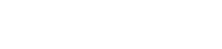This is a direct consequence of the fact that any integer divided by one is equal to that integer: it doesn’t change a number to multiply or divide it by one.  Thus, any integer can be written as a fraction, and the reciprocal would simply be one over the integer: the reciprocal of 15 is 1/15.  Thus, we can do all combinations.This last one was left as an improper fraction (i.e. the numerator is bigger than the denominator).  This is fine for now.  In the next post, I will discuss the issue of improper fractions vs. mixed numerals.

## Caveat #2: canceling

In all the examples in this discussion so far, I have given only examples in which canceling would not be an issue.  I avoided it so far because it is such an important topic that it deserves its own treatment.

We have already discussed the fact that any number over itself divides to one.  We already discussed how we can use that fact to construct fractions such as 3/3 or 17/17 that have a numerical value of one: such fractions play a role in creating common denominators.

Many times, especially if the numerator and denominator are larger numbers, we can cancel a common factor in the numerator and denominator of the same fractions.  This is called simplifying.  When we simplify fully, so that no more simplifying is possible, this is called putting the fraction is simplest terms.  It is an extremely valuable mathematical habit to put into simplest terms every fraction that crosses your path!!

For example, the fraction 15/25 has a common factor of 5 in both the numerator and denominator.  When we cancel the factor of 5, we are left with 2/3, a simplified fraction.

Some people think that canceling means that factors simply “go away”—in fact, as reprehensible as this is, some math teachers even use this poor language.  Nothing in mathematics simply “goes away.”  Cancelling is fundamentally an act of division.  This becomes very significant when we have to simplify a fraction such as 8/56.  Clearly, both numerator and denominator have a factor of 8, because 56 = 8*7.  Clearly, when the 8 in the denominator cancels, we are left with a 7 in the denominator.  What happens in the numerator?  Folks holding the erroneous “goes away” position might get confused here by the nothingness that they think results in the numerator once the 8 “goes away.’  In fact, what has happened is division, and 8 divided by 8 is 1.  We are left with a one in the numerator and a seven in the denominator: the fraction simplifies to 1/7.

We can simplify in a single fraction, but most of the individual fractions you will be given on the Praxis Core Mathematics Test already will be in simplest form.  Canceling takes on a much larger role in the multiplication and division of fractions.

Here we run into another poisonous term of which some math teachers are fond: cross-canceling.  I will discuss below why I think this term is detrimental to understanding fractions.  Here, we simply don’t need it.  Suppose a problem resulted in the following multiplication: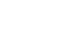What can we cancel?  The rule is simple.  When we are multiplying fractions, we can cancel any numerator with any denominator!  For example we can cancel the factor of 7 in common between the 63 and the 49, simplifying the fraction on the right.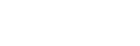Now, we can cancel that other 7 in the denominator with the factor of 7 in 35 (remember that we are left with a one after we cancel the 7).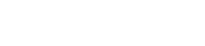Now, we can cancel the factor of 9 in the 27 with the 9, and then we can complete the multiplication.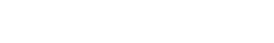Another beautiful improper fraction: for now, we will leave it in that form.  Notice that, once we canceled, the multiplication was ridiculously easy to perform.  Notice that if we had begun by multiplying the uncanceled original numbers, we would have gotten huge numbers, 2205 in the numerator and 1323 in the denominator—good luck canceling common factors from those gargantuans!  Choosing to multiply uncanceled numbers is like banging in nails with your head: it is a spectacularly bad idea!  One of the most productive mathematical habits you can cultivate is: always cancel completely before you multiply.   The value of this should be evident from the multiplication above.

## Caveat #3: proportions

Another word for a fraction is a ratio.  The two words mean the same thing mathematically, although ratio is more often used to denote a fraction of some real world or geometric quantities.  A proportion is simply an equation of the form [fraction] = [fraction].  Again, proportions are often set up to solve all kind of real world or geometric problems.  Here, I will just discuss the pure mathematics of proportions themselves, not their myriad real world applications.

For any proportion, a 100% bonafide all-the-time move is something called cross-multiplication.  It works like this: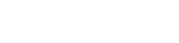Each numerator is multiplied by the denominator on the opposite side.  This is a totally legitimate, 100% correct move, and the advantage of it is that it eliminates fractions.  If there is an algebraic variable somewhere in the proportion, then we go from an algebra problem with fractions to one with no fractions: always a step toward simplification!

As with ordinary multiplication of fractions, it’s good to cancel before you multiply.  The question is: exactly what are we allowed to cancel in a proportion?  Of course, we can always do up-down cancellation within either single fraction.  Let’s call that vertical cancellation: up-and-down in a single fraction.

We also are allowed to cancel a common factor in both numerators: this is the equivalent of dividing both sides by the same number.  We can also cancel a common factor in both denominator: this is the equivalent of multiplying both sides by the same number.  Let’s call this horizontal canceling.  These are the legitimate forms of canceling in a proportion:

All good so far, but a great deal of confusing arises from the deleterious idea of cross-canceling.  You see, the proper domain of this suspect idea is the multiplication of fractions, and once there’s an equal sign between two fractions instead of a multiplication sign, all the rules are different.  Folks who learn cross-canceling mechanically mistakenly apply it to the proportion situation, and the very name seems to reinforce this mechanical misapprehension.  The following kind of canceling is 100% illegal and never valid in proportions.

Student who diligent learned cross-canceling are sometimes astounded to learn that this kind of canceling is illegal in a proportion, while the horizontal canceling above is legal.  If this is your reaction, then your understanding has been damaged by the poisonous influence of the idea of cross-canceling.

## Summary

If you had any moments of “aha” while you were reading this article, you may want to give the practice problems at the top another look before reading the explanations below.  The final article in this series will explore the issue of improper fractions vs. mixed numerals.## Practice problem explanations

1) Think of the 36 in the numerator as 36/1, and we are dividing two fractions.  This means we multiply the numerator fraction, 36/1, by the reciprocal of the denominator.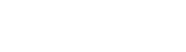2) This one contains a trap for people who mistakenly think that the idea of “cross-canceling” applies to proportions.  We cannot cancel diagonally in a proportion.  We can cancel a factor of 3 in the two numerators before we cross-multiply: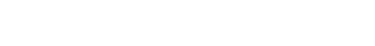By the way, sign up for our 1 Week Free Trial to try out Magoosh Praxis Prep!